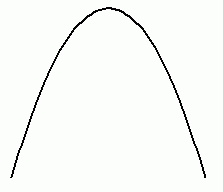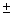From: Mike, a secondary school student A bridge over a river is supported by a parabolic arch...arch is 200 m wide at water level...the maximum height of the arch is 80 m..what is the height of the arch measured from a point on the water 40m from the center of the arch? Hi Mike. I've drawn a parabolic arch:You know that the arch is 80m high. If we choose to draw this on a graph or represent it in an equation, we may as well call the peak of the arch the vertex and put it on the y axis at position (0, 80). That means the ground is along the x = 0 line (the x axis). The distance between the feet of the arch is 200 m, so that means that the feet are at positions (100, 0). Knowing this, you can create an equation for the parabola. Remember the standard form of a parabola is y = a(x - h)2 + k. where (h, k) represents the vertex of the parabola and a controls the shape and vertical direction. You need to solve for a by substituting in one of the points (100, 0) in for x and y. This gives you the equation of the parabola. Since the center of the arch is on the y axis, 40 m from the center of the arch is just x =40 (you can choose either since the parabola is symmetric). So plug 40 in for x and get y, the height of the parabolic arch at this point. Hope this helps,Stephen La Rocque.